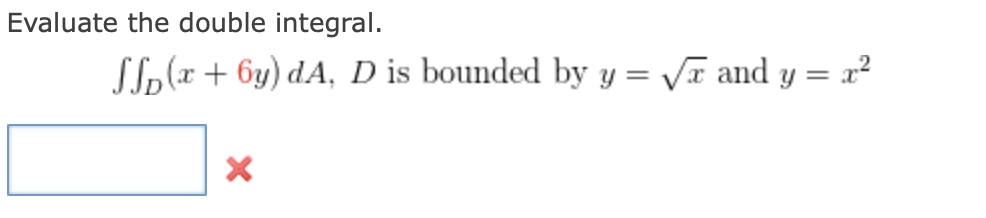Home / Expert Answers / Calculus / evaluate-the-double-integral-d-x-6y-da-d-is-bounded-by-y-x-and-y-x2-pa502

# (Solved): Evaluate the double integral. D(x+6y)dA,D is bounded by y=x and y=x2 ...Evaluate the double integral. is bounded by and

We have an Answer from Expert